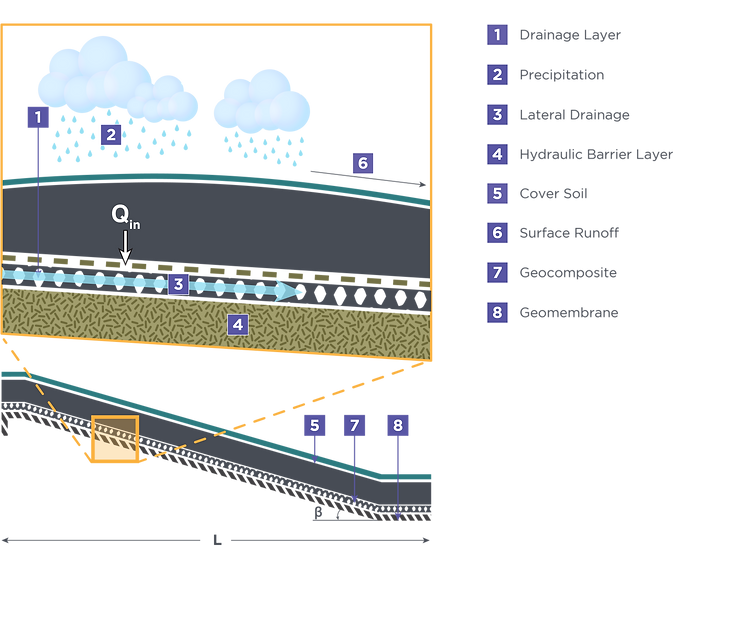# DESIGN METHODS & CONCEPTS

The final cover geocomposite is relatively close to the surface of the landfill and is therefore directly affected by short-term inputs from precipitation. The geocomposite is typically overlain by approximately 2 ft (0.6 m) of protective and vegetative soil. The properties of this soil layer can significantly influence how much precipitation impinges on the drainage layer. Thiel and Stewart  describe a relatively simple and conservative method of estimating the amount of liquid that may percolate into the drainage layer. Their approach has since been labeled the “unit gradient” method. The basis for this method is that for the critical condition it can be assumed that the cover soil is saturated, and water from continued rains will percolate vertically through the cover soil. Since the head on top of the cover soil is practically zero (due to runoff), the gradient through the cover soil is unity. The infiltration rate is considered to be equal to the permeability of cover soil (k        ). Therefore, Darcy’s law gives the inflow percolation as (see also Figure 4.1):

# cover

EQUATION 4.1

WHERE

Q     = inflow percolation rate (m  /sec)

k           = permeability of cover soil

i     = inflow gradient = 1

A = area (m   )

# cover

If we examine a unit width of the cover slope, the area would be equal to the slope length (or distance between drainage outlets), L, times the unit width. Therefore,

# cover

EQUATION 4.2

WHERE

Q     = inflow percolation rate (m  /sec)

k           = permeability of cover soil

L = length / distance between drainage outlets

# cover

If we desire that all flow that infiltrates down to the drainage geocomposite is carried entirely by the geocomposite (i.e., the head above the geomembrane is less than or equal to the thickness of the geocomposite), then the limiting flow condition at the downstream end of the geocomposite (per unit width) would be:

# out               req

EQUATION 4.3

WHERE

Q       = the flow rate coming out of the drainage geocomposite (m  /sec-m)

k           = in-plane permeability of the geocomposite

i       = the gradient of the flow within geocomposite = sinβ

A = area (m   )

t = geotextile thickness (cm)

θ       = the transmissivity of the geocomposite (m  /sec-m)

β = the slope angle

# 2

FIGURE 4.1 DISPOSITION OF PRECIPITATION IN A TYPICAL FINAL COVER SYSTEMBy establishing that Q     = Q       , an equation can be written solving for the required transmissivity of the geocomposite, as follows:

# req

EQUATION 4.4

WHERE

θ       = the transmissivity of the geocomposite (m  /sec-m)

k           = permeability of cover soil

L = length / distance between drainage outlets

β = the slope angle

# cover

Examining Equation 4.4, we see that required transmissivity is a function of the inflow percolation, slope length, and slope angle.  A typical underdrain outlet design for a bench location is presented in Figure 4.2.FIGURE 4.2 TYPICAL UNDERDRAIN OUTLET AT BENCH

The allowable transmissivity of geocomposite is:

EQUATION 4.5

WHERE

# 2

θ          = allowable transmissivity of geocomposite

θ       = the transmissivity of the geocomposite (m  /sec-m)

FS factor of safety for permeability

The ultimate 100 hour transmissivity test value is calculated as follows:

EQUATION 4.6

# EQUATION SHEET

Choose input parameter values

SOLUTION